# Math in Focus Grade 5 Chapter 5 Practice 4 Answer Key Inequalities and Equations

Go through the Math in Focus Grade 5 Workbook Answer Key Chapter 5 Practice 4 Inequalities and Equations to finish your assignments.

## Math in Focus Grade 5 Chapter 5 Practice 4 Answer Key Inequalities and Equations

Complete with >, <, or =.

Question 1.
For y = 3, 6y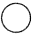11.
Here y = 3
6y = 6 x 3 = 18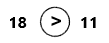Explanation:
We know that y = 3, multiply 3 with 6 the product is 18. Place 18 instead of 6y. Now compare both the numbers 18 and 11. The number 18 is greater than 11. So, place’ >’ symbol in the above circle.

Question 2.
For y = 6, 6y36.
Here y = 6
6y = 6 x 6 = 36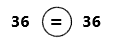Explanation:
We know that y = 6, multiply 6 with 6 the product is 36. Place 36 instead of 6y. Now compare both the numbers 36 and 36. The number 36 is equal to 36. So, place ‘=’ symbol in the above circle.

Question 3.
For y = 4, 6y26.
Here y = 4
6y = 6 x 4 = 24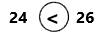Explanation:
Here we know that y = 4, multiply 4 with 6 the product is 24. Place 24 instead of 6y. Now compare both the numbers 24 and 26. The number 24 is less than 26. So, place ‘< ‘ symbol in the above circle.

Question 4.
For y = 5, 6y24.
Here y = 5
6y = 6 x 5 = 30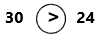Explanation:
We know that y = 5, multiply 5 with 6 the product is 30. Place 30 instead of 6y. Now compare both the numbers 30 and 24. The number 30 is greater than 24. So, place ‘>’ symbol in the above circle.

Complete with >, <, or = for x = 8.

Question 5.
3x20
X = 8
3X = 3 x 8 = 24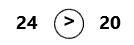Explanation:
We know that X = 8, multiply 8 with 3 the product is 24. Place 24 instead of 3X. Now compare both the numbers 24 and 20. The number 24 is greater than 20. So, place ‘>’ symbol in the above circle.

Question 6.
5x + 545
X = 8
5X = 5 x 8 = 40
5X + 5 = 40 + 5 = 45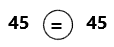Explanation:
We know that X = 8, multiply 8 with 5 the product is 40. Place 40 instead of 5X. Add 40 with 5 the sum is 45. Now compare both the numbers 45 and 45. The number 45 is equal to 45. So, place ‘=’ symbol in the above circle.

Question 7.
2x – 9x – 1
X = 8
2X = 2 x 8 = 16
16 – 9 = 7
8 – 1 = 7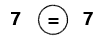Explanation:
We know that X = 8, The given expressions are 2X – 9 and X – 1. Place X value in the expressions. multiply 8 with 2 the product is 16. Place 16 instead of 2X. Subtract 9 from 16 the difference is 7. Subtract 1 from 8 the difference is 7. Now compare both the numbers 7 and 7. The number 7 is equal to 7. So, place ‘=’ symbol in the above circle.

Question 8.
12 – xx ÷ 2
X = 8
12 – x = 12 – 8 = 4
x ÷ 2 = 8 ÷ 2 = 4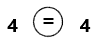Explanation:
We know that X = 8, The given expressions are 12 -X and X ÷ 2. Place x value in the expressions. Subtract 8 from 12 the difference is 4. Place 4 instead of 12 – X. Divide 8 by 2 the result is 4. Place 4 instead of X ÷ 2. Now compare both the numbers 4 and 4. The number 4 is equal to 4. So, place ‘=’ symbol in the above circle.

Solve each equation.

Example
x – 5 = 5
x – 5 + 5 = 5 + 5
x = 10
x = 10

Question 9.
2a + 4 = 10
a = ____
2a + 4 = 10
2a = 10 – 4
2a = 6
a = 6/2
a = 3

Question 10.
5b – 13 = 17
b = ____
5b – 13 = 17
5b = 17 + 13
5b = 30
b = 30/5
b = 6

Question 11.
2m – 3 = m
m ______
2m – 3 = m
2m + m = 3
3m = 3
m = 3/3
m = 1

Question 12.
12n + 7 = 8n + 15
n = ____